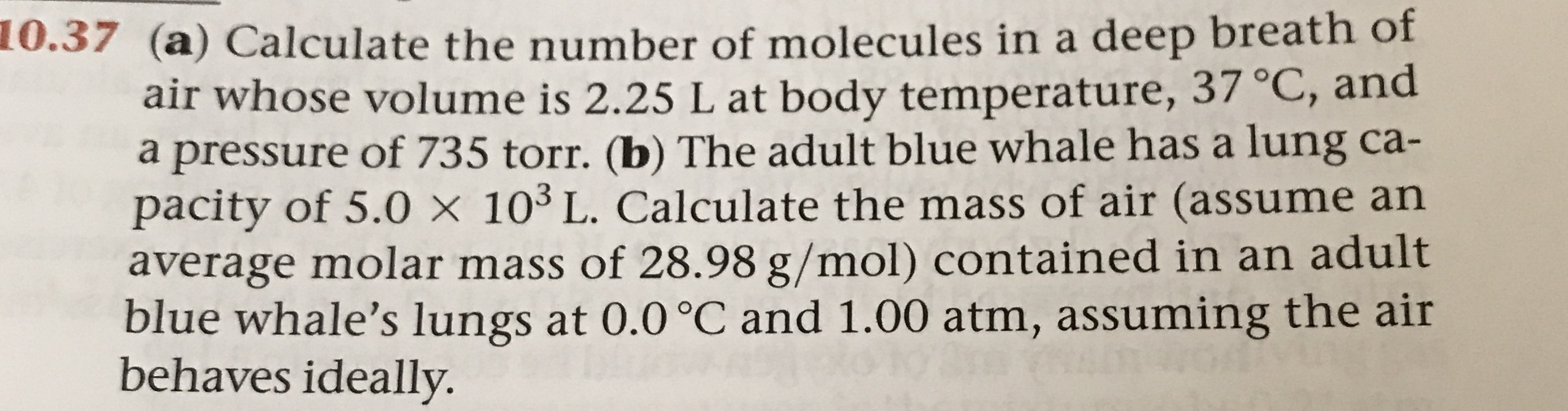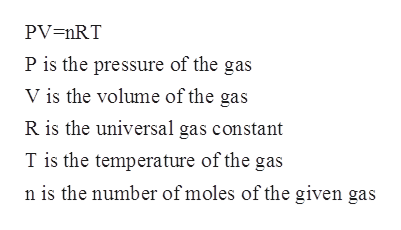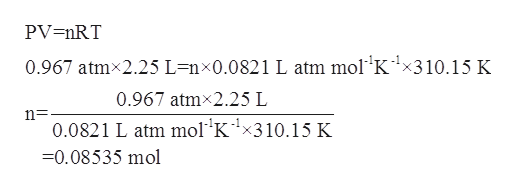# 0.37 (a) Calculate the number of molecules in a deep breath ofair whose volume is 2.25 L at body temperature, 37 °C, anda pressure of 735 torr. (b) The adult blue whale has a lung ca-pacity of 5.0 x 103 L. Calculate the mass of air (assume anaverage molar mass of 28.98 g/mol) contained in an adultblue whale's lungs at 0.0 °C and 1.00 atm, assuming the airbehaves ideally.

Question
61 viewshelp_outlineImage Transcriptionclose0.37 (a) Calculate the number of molecules in a deep breath of air whose volume is 2.25 L at body temperature, 37 °C, and a pressure of 735 torr. (b) The adult blue whale has a lung ca- pacity of 5.0 x 103 L. Calculate the mass of air (assume an average molar mass of 28.98 g/mol) contained in an adult blue whale's lungs at 0.0 °C and 1.00 atm, assuming the air behaves ideally. fullscreen
check_circle

Step 1

The ideal gas equation is known as the general gas equation and is a equation of state for a given hypothetical gas. The equation is given by:help_outlineImage TranscriptionclosePV=nRT P is the pressure of the gas V is the volume of the gas R is the universal gas constant T is the temperature of the gas n is the number of moles of the given gas fullscreen
Step 2

The pressure of the air is given to be 735 Torr. It can be converted to atm as follows:

Step 3

The volume of the air is given to be 2.25 L.

The temperature is given to be 37oC= 310.15 K.

The gas constant is taken to be 0.0...help_outlineImage TranscriptionclosePV =nRT 0.967 atm 2.25 L=nx0.0821 L atm mol'K1x310.15 K 0.967 atm 2.25 L n= 0.0821 L atm mol K1x310.15 K 0.08535 mol fullscreen

### Want to see the full answer?

See Solution

#### Want to see this answer and more?

Solutions are written by subject experts who are available 24/7. Questions are typically answered within 1 hour.*

See Solution
*Response times may vary by subject and question.
Tagged in

### Physical Chemistry